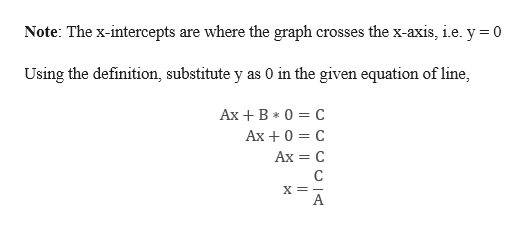# show that the x-intercept of the graph of Ax+By=C is (c/a,0)

Question
61 views

show that the x-intercept of the graph of Ax+By=C is (c/a,0)

check_circle

Step 1

Given equation,

Step 2

To find the x intercept of the ...help_outlineImage TranscriptioncloseNote: The x-intercepts are where the graph crosses the x-axis, ie. y 0 Using the definition, substitute y as 0 in the given equation of line, Ax B 0 C Ах +0 3 с Ах — С C X=- fullscreen

### Want to see the full answer?

See Solution

#### Want to see this answer and more?

Solutions are written by subject experts who are available 24/7. Questions are typically answered within 1 hour.*

See Solution
*Response times may vary by subject and question.
Tagged in

### Equations and In-equations